AnyLogic

# negativeBinomial

 Probability mass function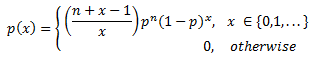Distribution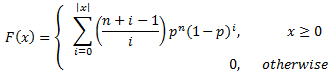Mean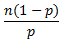Variance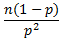The Negative Binomial distribution is a discrete distribution bounded on the low side at 0 and unbounded on the high side. The Negative Binomial distribution gives the number of failures before the nth success in a sequence of independent Bernoulli trials with probability p of success on each trial.

The Negative Binomial distribution has many uses; some occur because it provides a good approximation for the sum or mixing of other discrete distributions. By itself, it is used to model accident statistics, birth-and-death processes, market research and consumer expenditure, lending library data, biometric data, and many others.

#### Examples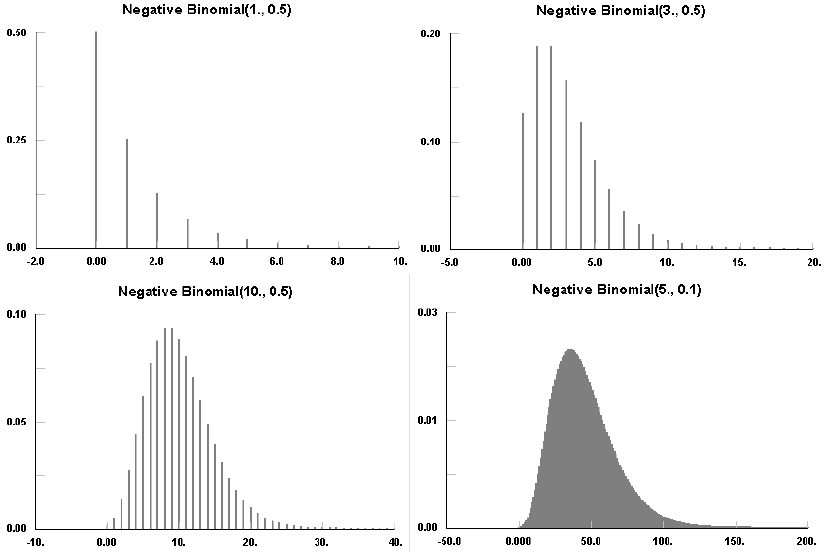### negativeBinomial(double p, double n)

Description
Generates a sample of the Negative Binomial distribution.
Parameters
Name Type of value Description
p double Probability of success on each trial.
n double Number of desired successes.
Result
Type Description
double The generated sample.

### negativeBinomial(double p, double n, java.util.Random r)

Description
Generates a sample of the Negative Binomial distribution using the specified random number generator.
Parameters
Name Type of value Description
p double Probability of success on each trial.
n double Number of desired successes.
r java.util.Random The random number generator.
Result
Type Description
double The generated sample.

This document includes content from the “Stat::Fit User’s Manual”. Copyright 2016 Geer Mountain Software Corp.